Search by Topic

Resources tagged with Algorithms similar to A Brief Introduction to Complex Numbers:

Filter by: Content type:
Age range:
Challenge level:

There are 20 results

Broad Topics > Decision Mathematics and Combinatorics > Algorithms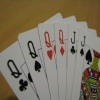Sorted

Age 14 to 18 Challenge Level:

How can you quickly sort a suit of cards in order from Ace to King?Method in Multiplying Madness?

Age 7 to 14 Challenge Level:

Watch our videos of multiplication methods that you may not have met before. Can you make sense of them?X Marks the Spot

Age 11 to 14 Challenge Level:

When the number x 1 x x x is multiplied by 417 this gives the answer 9 x x x 0 5 7. Find the missing digits, each of which is represented by an "x" .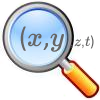The Best Square

Age 14 to 18 Challenge Level:

How would you judge a competition to draw a freehand square?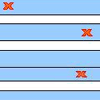Stretching Fractions

Age 14 to 16 Challenge Level:

Imagine a strip with a mark somewhere along it. Fold it in the middle so that the bottom reaches back to the top. Stetch it out to match the original length. Now where's the mark?Unusual Long Division - Square Roots Before Calculators

Age 14 to 16 Challenge Level:

However did we manage before calculators? Is there an efficient way to do a square root if you have to do the work yourself?Zeller's Birthday

Age 14 to 16 Challenge Level:

What day of the week were you born on? Do you know? Here's a way to find out.Triangle Incircle Iteration

Age 14 to 16 Challenge Level:

Keep constructing triangles in the incircle of the previous triangle. What happens?Funny Factorisation

Age 11 to 14 Challenge Level:

Using the digits 1 to 9, the number 4396 can be written as the product of two numbers. Can you find the factors?Skeleton

Age 11 to 14 Challenge Level:

Amazing as it may seem the three fives remaining in the following `skeleton' are sufficient to reconstruct the entire long division sum.Tis Unique

Age 11 to 14 Challenge Level:

This addition sum uses all ten digits 0, 1, 2...9 exactly once. Find the sum and show that the one you give is the only possibility.Kids

Age 11 to 14 Challenge Level:

Find the numbers in this sum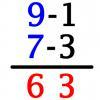Vedic Sutra - All from 9 and Last from 10

Age 14 to 16 Challenge Level:

Vedic Sutra is one of many ancient Indian sutras which involves a cross subtraction method. Can you give a good explanation of WHY it works?Alphabet Soup

Age 11 to 14 Challenge Level:

This challenge is to make up YOUR OWN alphanumeric. Each letter represents a digit and where the same letter appears more than once it must represent the same digit each time.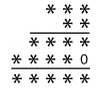Long Multiplication

Age 11 to 14 Challenge Level:

A 3 digit number is multiplied by a 2 digit number and the calculation is written out as shown with a digit in place of each of the *'s. Complete the whole multiplication sum.Slippy Numbers

Age 11 to 14 Challenge Level:

The number 10112359550561797752808988764044943820224719 is called a 'slippy number' because, when the last digit 9 is moved to the front, the new number produced is the slippy number multiplied by 9.Medal Muddle

Age 11 to 14 Challenge Level:

Countries from across the world competed in a sports tournament. Can you devise an efficient strategy to work out the order in which they finished?Peaches in General

Age 14 to 16 Challenge Level:

It's like 'Peaches Today, Peaches Tomorrow' but interestingly generalized.Tournament Scheduling

Age 11 to 16

Scheduling games is a little more challenging than one might desire. Here are some tournament formats that sport schedulers use.On What Day Did it Happen?

Age 5 to 14

Read this article to find out the mathematical method for working out what day of the week each particular date fell on back as far as 1700.